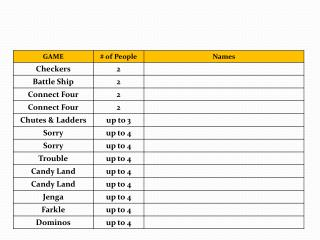Download PresentationStoichiometry

# Stoichiometry - PowerPoint PPT PresentationDownload Presentation## Stoichiometry

- - - - - - - - - - - - - - - - - - - - - - - - - - - E N D - - - - - - - - - - - - - - - - - - - - - - - - - - -
##### Presentation Transcript

1. Stoichiometry Test review Let’s find out if we can be ready for a test on Thursday 3/06!

2. Games!

3. At each question, each team mate with a correct answer gets to take their turn. • If you need an explanation, ask your partner for help. If that doesn’t work, ask me to go over it before the next question! • Keep a record of points scored and games won. • Most games won, or person closest to winning your game at the bell gets tickets!

4. Name three of the seven diatomic elements.

5. H2 F2 Cl2 Br2 I2 O2 N2

6. What is the molar mass of Al(OH)3?

7. 26.98 + (3 x 16) + (3 x 1) = 77.98 g/mol

8. What is the name of the conversion factor that lets us go from moles to grams?

9. Molar mass

10. What is the name of the conversion factor that lets us go from moles to molecules?

12. What is the name of the conversion factor that lets us go from volume to moles?

13. Molar volume

14. What is the name of the conversion factor that lets us go from moles of one compound to moles of another compound?

15. Mole ratio

16. What is the mole ratio of TNT to carbon monoxide in the following equation? C7H5N3O6 CO + C + H2O + N2

17. 2 mole TNT : 7 mole CO

18. What is the formula of sodium carbonate?

19. Na2 CO3

20. What is the molar mass of sodium carbonate?

21. Na2 CO3 • 23+23+12+16+16+16= 106g/mol

22. What is the molar mass of iron (III) sulfate

23. Fe2(SO4)3 • 55.9+55.9+ 3(32.1) + 12(16)=400.1 g/mole

24. How many moles of iron (III) sulfate in 44.5g iron (III) sulfate? The molar mass is 400.1 g.

25. 44.5g Fe2(SO4)3 * 1 mole/400.1g = .11 moles Fe2(SO4)3

26. How many grams potassium chloride are in 14.6 moles potassium chloride? 1 mole = 74.5 g

27. =14.6 moles KCl* 74.5 g/mole KCl = 1087.7g KCl

28. How many grams of iron (III) carbonate are found in 5.46 moles iron (III) carbonate?

29. 5.46 moles Fe2(CO3)3 x 291.7 g Fe2(CO3)3/1 mole Fe2(CO3)3 =1592.68 gFe(CO3)3

30. How many moles of sodium carbonate are in 10.9 g sodium carbonate? Molar mass sodium carbonate 106 g

31. 10.9 g Na2CO3 x 1 mole Na2CO3/106g =0.103 moles

32. What is the molar ratio of iron(III) sulfate to sodium sulfate when iron(III) sulfate reacts with sodium carbonate? Fe2(SO4)3 + 3Na2CO3 Fe2(CO3)3 + 3Na2SO4

33. Fe2(SO4)3 + 3Na2CO3 Fe2(CO3)3 + 3Na2SO4 1 mole Fe2(SO4)3 = 3 moles Na2SO4

34. If 10 moles iron (III) sulfate reacts, how many moles sodium carbonate will form?Fe2(SO4)3 + 3Na2CO3 Fe2(CO3)3 + 3Na2SO4

35. Fe2(SO4)3 + 3Na2CO3 Fe2(CO3)3 + 3Na2SO4 10 moles Fe2(SO4) x 3 moles Na2CO3/1mole Fe2(SO4)3 = 30 molesNa2CO3

36. If 15 g oxygen is reacted with hydrogen, then how many moles water will be produced?

37. 2H2 + O2 2H2O 15 gO2 X 1moleO/32 gO X 2moles H2O/moleO = 0.94 moles water

38. If 46 g sodium metal reacts completely, then how many moles chlorine gas will be required to make sodium chloride?

39. 2Na + Cl2 2NaCl 46gNa X 1moleNa/23gNa X 1mole Cl2/2molesNa = 1 mole Cl2

40. If 100 g sodium chloride reacts completely with barium, then how many grams sodium metal will be obtained? 2NaCl + Ba  BaCl2 + 2Na

41. 2NaCl + Ba  BaCl2 + 2Na 100gNaCl x 1mole NaCl/58.4 g NaCl x 2 mole Na/2 moles NaCl X 23 g/1mole Na =39.32 g Na

42. How many liters fluorine gas in .87 moles of fluorine gas? • 1 mole gas at STP (standard temperature and pressure, O degrees C and 1 atm pressure) = 22.4 L gas

43. .87moles F2 * 22.4 L/mole =19.52L F2

44. How many grams of oxygen gas are in .69L of oxygen gas? (22.4 L/1 mole)

45. .69 L O2 x 1 mole O2 / 22.4 L x (16+16)g/1mole O2 = .99g

46. 2 H2S + 3 O2 2 SO2+ 2 H2OHow many moles of H2S are required to form 8.20 moles of SO2?

47. 8.2 mol • 8.2 mole SO2 x (2mol H2S/2 mol SO2)

48. 2 NaClO3 2 NaCl + 3 O2 How many molecules of oxygen are produced when 80.0 grams of sodium chloride are produced?

49. 1.23 x 1024 molecules (80gA) x(1molA/58.4gA)x (3mol B/2molA)x(6.02x1023molecB)